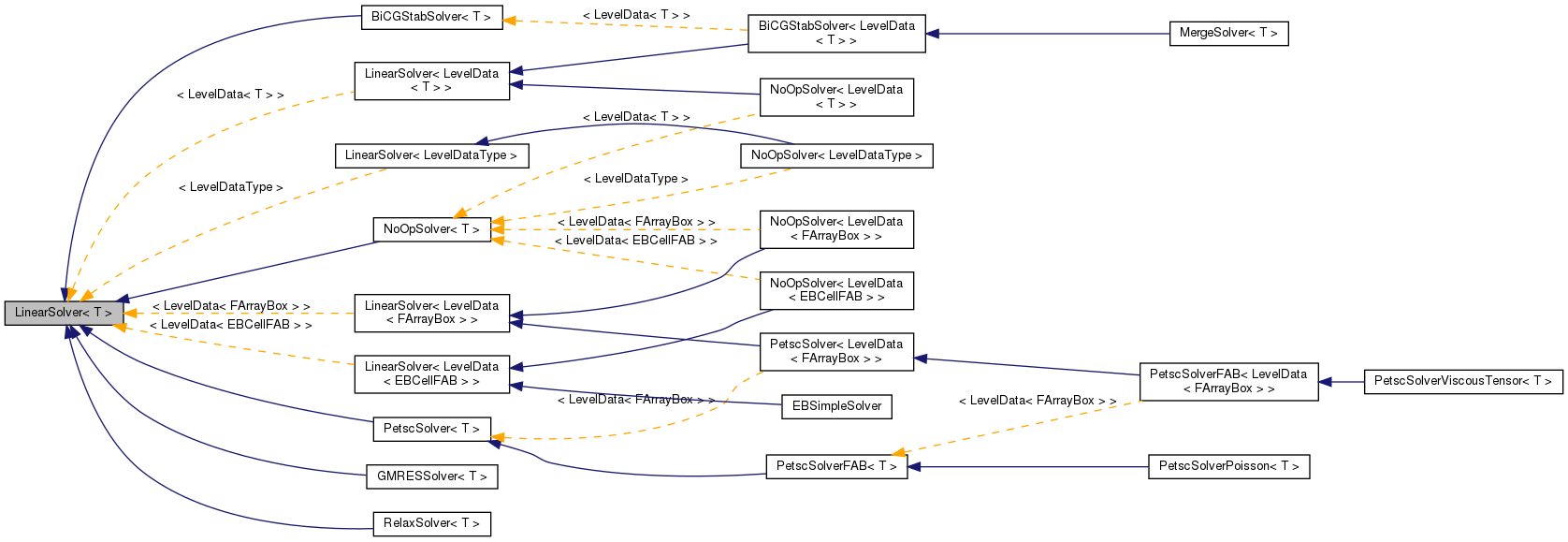Chombo + EB  3.0
LinearSolver< T > Class Template Referenceabstract

`#include <LinearSolver.H>`

Inheritance diagram for LinearSolver< T >:[legend]

## Public Member Functions

virtual ~LinearSolver ()

virtual void setHomogeneous (bool a_homogeneous)=0

virtual void define (LinearOp< T > *a_operator, bool a_homogeneous=false)=0

virtual void solve (T &a_phi, const T &a_rhs)=0

virtual void setConvergenceMetrics (Real a_metric, Real a_tolerance)
Set a convergence metric, along with solver tolerance, if desired. More...

## Detailed Description

### template<class T> class LinearSolver< T >

Generic linear solver template. BiCGStab and others are built on top of this.

## ◆ ~LinearSolver()

template<class T>
 virtual LinearSolver< T >::~LinearSolver ( )
inlinevirtual

## ◆ setHomogeneous()

template<class T>
 virtual void LinearSolver< T >::setHomogeneous ( bool a_homogeneous )
pure virtual

reset whether the solver is homogeneous.

## ◆ define()

template<class T>
 virtual void LinearSolver< T >::define ( LinearOp< T > * a_operator, bool a_homogeneous = `false` )
pure virtual

Define the operator and whether it is a homogeneous solver or not. The LinearSolver does not take over ownership of this a_operator object. It does not call delete on it when the LinearSolver is deleted. It is meant to be like a late-binding reference. If you created a_operator with new, you should call delete on it after LinearSolver is deleted if you want to avoid memory leaks.

## ◆ solve()

template<class T>
 virtual void LinearSolver< T >::solve ( T & a_phi, const T & a_rhs )
pure virtual

Solve L(phi) = rhs (phi = L^-1 (rhs)).

## ◆ setConvergenceMetrics()

template<class T>
 virtual void LinearSolver< T >::setConvergenceMetrics ( Real a_metric, Real a_tolerance )
inlinevirtual

Set a convergence metric, along with solver tolerance, if desired.

Default implementation does nothing, since there are probably cases (liked direct solves), where this has no real meaning.

The documentation for this class was generated from the following file: